# Naive Bayes Implementation with Python

## Introduction

The word 'Bayes' in Naive Bayes comes from Bayes Theorem from probability theory. The Bayes theorem states that given an event, A occurred. What is the probability of occurrence of another event B, given that A has already happened. So, there is a condition between the two events. They are not Independent.

``Bayes Theorem: P(B/A)  =  P(A/B)  P(A)/P(B)``

Let's consider an example where we have 100 emails, 25 are spam, and 75 are non-spams.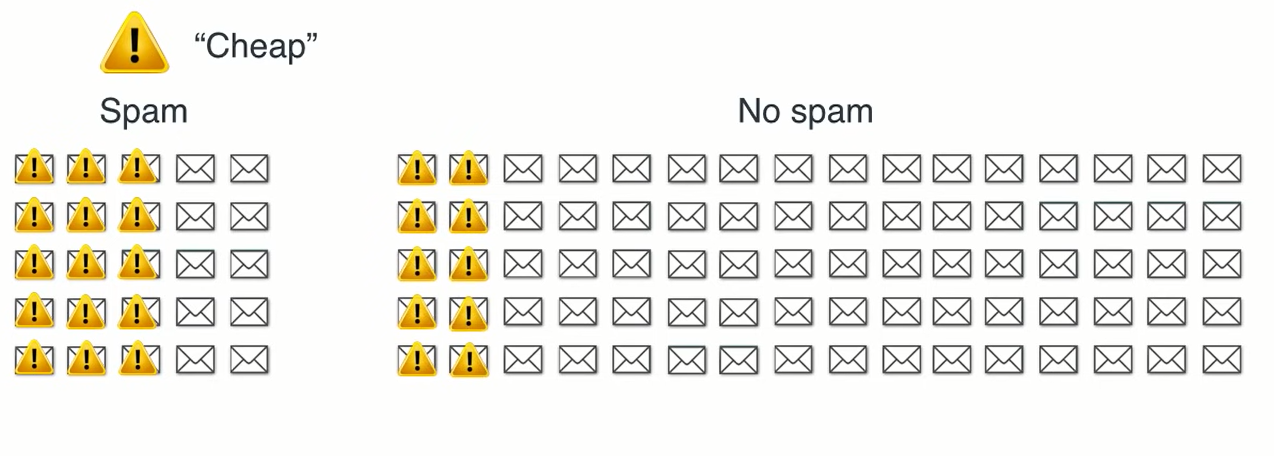20 out of 25 spam emails contain the word "cheap," and  5 out of 75 non-spam emails have "cheap."  Given a mail containing the word "cheap," what is the probability of it being spam?

Consider an event A = mail contains the word cheap.

Event B = mail is spam.

P(A)= 25/100 = 0.25

P(B)= 25/100 = 0.25

P(A/B)= 20/25 = 0.8

Therefore,  P(B/A)  = 0.8 x 0.25/0.25 = 0.8

So, there is an 80% chance that the mail is spam.

This is how the spam detector works.

The word 'Naive' in Naive Bayes indicates that the features in the datasets are Independent of each other, i.e., the occurrence of an event does not affect the occurrence of the other.

## Implementation of Titanic crash survival

I have downloaded the dataset in CSV format from the Kaggle website and loaded the same file into a pandas data frame into google-colab. Now, we are going to do some data exploration first.

``````import pandas as pd

Output: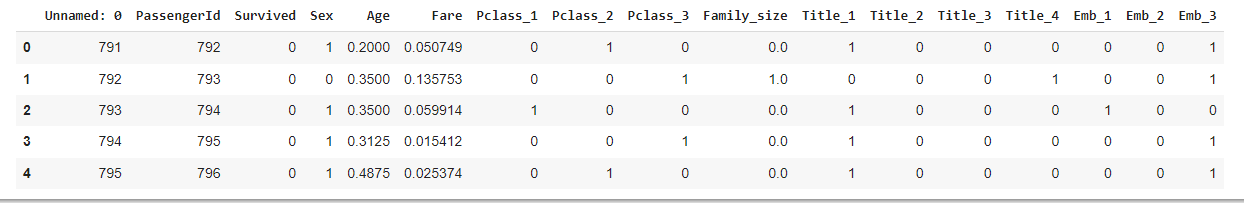df.head(5) returns the first five rows of the dataset. But, some of the features/columns are not relevant and probably don't impact the target variable. So we have to drop those features.

``df.drop(['Unnamed: 0','PassengerId','Fare','Sex','Family_size','Title_1','Title_2','Title_3','Title_4','Emb_1','Emb_2','Emb_3'], axis='columns', inplace=True)``

``df.head(10)``

Output: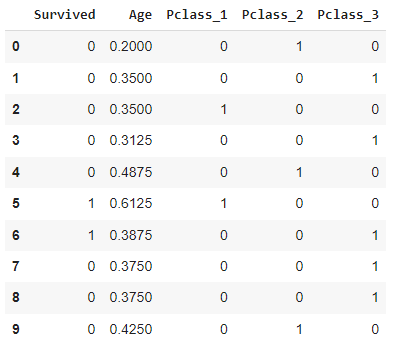There is a target variable(Independent variable) that I want to separate into different series, and the rest of the other features will go to some input(Dependent variable) series. I am dropping the target feature from the input.

``````target = df.Survived
inputs = df.drop(['Survived'],axis='columns')``````

Target looks like this: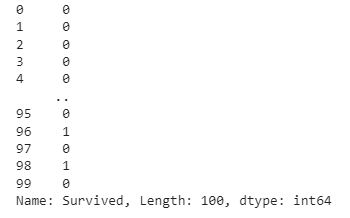Inputs looks like this: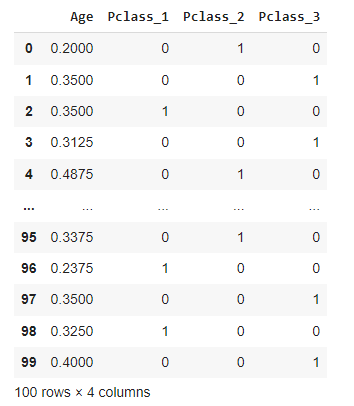Now, I will find whether there are any NAN numbers in the columns.

``inputs.columns[inputs.isna().any()]``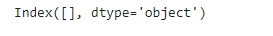This means we don't have any NAN value; otherwise, I had to replace those by the mean of those columns.

Now, I will choose the SKlearn train, test, split method to split the dataset into training and testing samples.

``````from sklearn.model_selection import train_test_split
x_train, x_test, y_train, y_test = train_test_split(inputs, target, test_size=0.2)``````

train data looks like this: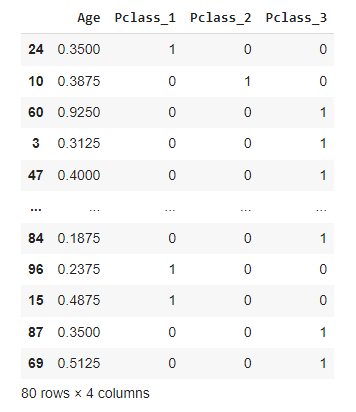Similarly, you can check for the rest.

Now, it's time to create the Naive Bayes model. There are various Naive Bayes classes, and I am going to use the Gaussian Naive Bayes Classifier. The use of different classifiers depends on the distribution of the data. You can read more about them here.

``````from sklearn.naive_bayes import GaussianNB
model = GaussianNB()``````

It's time to train the model using the fit function and check the training accuracy using the score method.

``````model.fit(x_train, y_train)
model.score(x_test, y_test)``````

Output: 0.7

The score is not very good, but okay. This means that 70% of the prediction by the model is accurate. More the datasets, the more accuracy scores will be.

Let’s check the first ten testing samples.

``y_test[0:10]``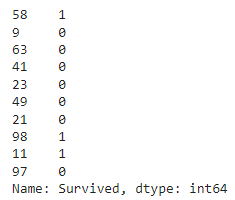And now predict the result using the model

``model.predict(x_test[0:10])``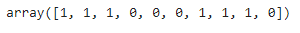The model is doing good, and the accuracy would increase with the number of datasets.

## FAQs

1. What is the reason that Naive Bayes has the word ‘Naive’ in it?
->Naive Bayes classifier assumes that a particular feature in a class is unrelated to the presence of any other feature.

2. What is an Independent Event?
-> Two or more events are said to be independent of each other if and only if the occurrence of any of them does not affect the other.

3. Name the technique used to avoid the zero probabilities while calculating probabilities using Naive Bayes.
-> Laplace Correction

4. What does a fit function do in Naive Bayes?
-> fit function trains the model with the datasets.

5. Given two events A and B, what does p(A/B) indicate?
-> slash ‘/’ means condition. p(A/B) means the probability of event A conditioned that event B has already occurred.

## Key Takeaways

This comes to the end of the Discussion on Naive Bayes and the Implementation. There are lots of Statistical processes besides the implementation of Naive Bayes. You could look at these here.

(must visit: Naive Bayes classifier: A friendly approach)